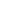# Free Class 9 Perimeter and area of different figures Worksheets

Download free printable Perimeter and area of different figures Worksheets to practice. With thousands of questions available, you can generate as many Perimeter and area of different figures Worksheets as you want.

## Sample Class 9 Perimeter and area of different figures Worksheet Questions

1.

The sides of a triangular field are 41 m, 40 m and 9 m. Find the number of flower beds that can be prepared in the field, if each flower bed needs space.

2.

The perimeter of an isosceles triangle is 32 cm. The ratio if the equal side to its base is 3 : 2. Find the area of the triangle.

3.

The sides of a quadrilateral ABCD are 6 cm, 8 cm, 12 cm and 14 cm (taken in order) respectively, and the angle between the first two sides is a right angle. Find its area.

4.

A rhombus shaped sheet with perimeter 40 cm and one diagonal 12 cm, is painted on both sides at the rate of ` 5 per . Find the cost of painting.

5.

Two side of a parallelogram are 10 cm and 7 cm. One of its diagonals is 13 cm. Find the area.

6.

The diagonals of a rhombus are 24 cm and 10 cm. Find its area and perimeter.

7.

Find the area of a right triangle whose sides containing the right angle are 5 cm and 6 cm.

8.

The area of an isosceles triangle is . If one of its equal side is 5cm. Find its base

9.

Find the area of a triangle, two sides of which are 8 cm and 11 cm and the perimeter is 32 cm.

10.

Find the cost of leveling a ground in the form of a triangle with sides 16 m, 12 m and 20 m at Rs. 4 per sq. meter.

Worksheets by UrbanPro

Our worksheets are designed to help students explore various topics, practice skills and enrich their subject knowledge, to improve their academic performance. Designed by Experts who have extensive experience and expertise in teaching a subject, these worksheets will improve your child's problem-solving skills and subject knowledge in a fun and interactive manner.
Check out our free customized worksheets across school boards, grades, subjects and levels of subject knowledge. You can download, print and share these worksheets with anyone, anywhere, anytime!

Get a custom worksheet to practice!

Select your topic & see the magic.

subjectSelect Chapter(s)

Chapters & Subtopics